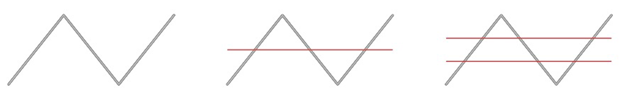Chapter PL, Problem 3P### Algebra and Trigonometry (MindTap ...

4th Edition
James Stewart + 2 others
ISBN: 9781305071742

#### Solutions

Chapter
Section### Algebra and Trigonometry (MindTap ...

4th Edition
James Stewart + 2 others
ISBN: 9781305071742
Textbook Problem
1 views

# Cutting up a Wire A piece of wire is bent as shown in the figure. You can see that one cut through the wire produces four pieces and two parallel cuts produce seven pieces. How many pieces will be produced by 142 parallel cuts? Write a formula for the number of pieces produced by n parallel cuts.To determine

To find:

The number of pieces is produced by 142 parallel cuts and to write a formula for number of pieces produced by n parallel cuts.

Explanation

Calculation:

Given that the one cut through the wire produces four pieces and two parallel cuts produce seven pieces.

From the given we know that sum of pieces increases by 3.

Now by this we can write the formula for the number of pieces produced by n parallel cuts is 3n+1.

Calculate the number of pieces is produced by 142 parallel cuts,

Here n

### Still sussing out bartleby?

Check out a sample textbook solution.

See a sample solution

#### The Solution to Your Study Problems

Bartleby provides explanations to thousands of textbook problems written by our experts, many with advanced degrees!

Get Started

## Chapter PL Solutions